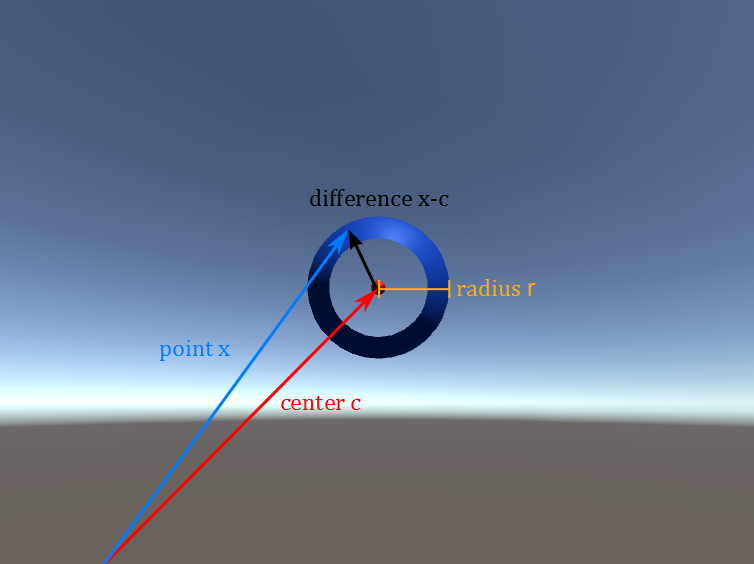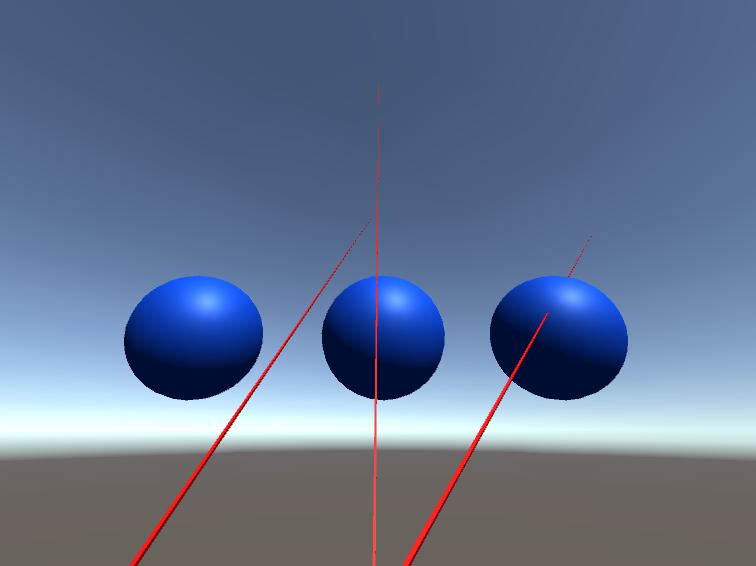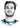# Howto Raytracer: Ray / Sphere Intersection Theory

Categories:

I will show you how to calculate the intersection point of a ray with a sphere.

A ray $$r(t)$$ can be represented by a point on the ray $$e$$ and the ray’s direction $$d$$. Any point on that ray can then be reached by walking $$t$$ times in the direction of $$d$$ from $$e$$ as $$r(t)=e + t d$$. The set $$R$$ of all points on the ray is then given by: $$R = {r(t) \mid t \in \mathbb{R}}$$

A sphere $$S$$ can be represented by its center point $$c$$ and its radius $$r$$. The set of all points $$x$$ whose distance from the center $$c$$ of the sphere equals the radius $$r$$, is by definition the set of points on the sphere: $$S = { ||x - c|| = r \mid x \in \mathbb{R}^3}$$. ($$||x||$$ denotes the length of a vector.)To find the intersection of the ray and the sphere now, we have to find the points that are in both sets. So we check if a point $$r(t)$$ on the ray also fullfills the distance equation of the sphere:

$$||r(t) - c|| = r$$

We now simply have to solve this equation. The way we do it is by rewriting the length of the vector as a dot product. For any vector $$x = (a,b,c)$$, its (euclidean) length is given by $$||x|| = \sqrt{a^2 + b^2 + c^2}$$. The dot product $$\cdot$$ of a vector $$x$$ with itself is the sum of its squared components $$x \cdot x = a^2 + b^2 + c^2$$.

So in the end we get the following relation:

\begin{aligned} ||r(t) - c|| = &\sqrt{(r(t) - c) \cdot (r(t) - c)} = r \\ &(r(t) - c) \cdot (r(t) - c) = r^2 \end{aligned}

The dot product has the same distributive and associative properties as the scalar multiplication, so you can do your maths the normal way:

\begin{aligned} (r(t) - c) \cdot (r(t) - c) &= r^2 \\ (e+td- c) \cdot (e+td - c) &= r^2 \\ e\cdot e + te\cdot d - e\cdot c &\\ +t e \cdot d + t^2 d\cdot d - t d\cdot c &\\ -c \cdot e - t c \cdot d + c \cdot c &= r^2 \end{aligned}

The dot product is commutative $$(x \cdot y = y \cdot x)$$, and after rearranging the terms according to our free paramter $$t$$ we end up with:

\begin{aligned} && t^2 d\cdot d & \\ &&+t 2(e \cdot d - c \cdot d) &\\ && +e\cdot e -2(e \cdot c) + c \cdot c &= r^2 \\ t^2 d\cdot d &+t 2(e \cdot d - c \cdot d) &+ e\cdot e -2(e \cdot c) + c \cdot c - r^2 &= 0 \\ t^2 d\cdot d &+t 2d\cdot(e - c) &+ (e-c) \cdot (e-c) - r^2 &= 0 \end{aligned}

Now we reduced it to a quadratic equation in $$t$$. Quadratic equations of the form $$at^2 + bt + c = 0$$ have the two solutions: $$t_{1,2} = \frac{-b\pm\sqrt{b^2-4ac}}{2a}$$

Applied to our equation we get:

$$t_{1,2} = \frac{-2d\cdot(e - c) \pm \sqrt{(2d\cdot(e - c))^2-4 d\cdot d ((e-c) \cdot (e-c) - r^2) }}{2 d\cdot d}$$

If and how many solutions exist depends on the discriminant, the term under the square root, $$D =(2d\cdot(e - c))^2-4 d\cdot d ((e-c) \cdot (e-c) - r^2)$$:

• $$D < 0$$: No solution. The ray misses the sphere
• $$D = 0$$: One solution. The ray touches the sphere in one point.
• $$D > 0$$: Two solution. The ray hits the sphere in two points, one entry hit and the exit hit on the other side

To get the intersections you calculate the $$t$$ values and then evaluate the ray $$r(t)$$.Left: No intersection
Middle: One intersection
Right: Two intersections

Finally some C# code that implements the ray / sphere intersection test:

public RayTracer.HitInfo Intersect(Ray ray)
{
RayTracer.HitInfo info = new RayTracer.HitInfo();

Vector3 eMinusS = ray.origin - center;
Vector3 d = ray.direction;
double discriminant = Math.Pow(2 * Vector3.Dot(d, eMinusS), 2) - 4 * Vector3.Dot(d, d) *
(Vector3.Dot(eMinusS, eMinusS) - Math.Pow(radius, 2.0f));

if (discriminant < -Mathf.Epsilon)
{   // 0 hits
return info;
}
else {      // there will be one or two hits
float front = -2.0f * Vector3.Dot(d, eMinusS);
float denominator = 2.0f * Vector3.Dot(d, d);
if (discriminant <= Mathf.Epsilon)
{   // 1 hit
info.time = (float)(front + Math.Sqrt(discriminant)) / denominator;  // does not matter if +- discriminant
}
else {  // 2 hits
float t1 = (float)(front - Math.Sqrt(discriminant)) / denominator;  // smaller t value
float t2 = (float)(front + Math.Sqrt(discriminant)) / denominator;  // larger t value
if (t2 < 0) // sphere is "behind" start of ray
{
return info;    // no hit
}
else {  // one of them is in front
if (t1 >= 0) info.time = t1; // return first intersection with sphere (usual case, smaller t)
else info.time = t2;        // return second hit (ray's origin is inside the sphere)
}
}
}

// if we are here, info.time has been set, otherwise the function would have returned
info.hitPoint = ray.GetPoint(info.time);
info.normal = (info.hitPoint - center).normalized;
return info;
}

# Hi, I'm Christoph Michel 👋I'm a , , and .

I mostly do and help small to mid-sized teams from all over the world 🌎 on both short and long-term projects on an independent contractor basis.

I strive for efficiency ⏱️ and therefore track many aspects of my life.